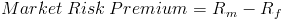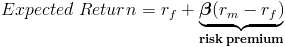The formula for risk premium, sometimes referred to as default risk premium, is the return on an investment minus the return that would be earned on a risk free investment. The risk premium is the amount that an investor would like to earn for the risk involved with a particular investment.

The US treasury bill (T-bill) is generally used as the risk free rate for calculations in the US, however in finance theory the risk free rate is any investment that involves no risk.

## Risk Premium of the Market

The risk premium of the market is the average return on the market minus the risk free rate. The term "the market" in respect to stocks can be connoted as an entire index of stocks such as the S&P 500 or the Dow. The market risk premium can be shown as:The risk of the market is referred to as systematic risk. In contrast, unsystematic risk is the amount of risk associated with one particular investment and is not related to the market. As an investor diversifies their investment portfolio, the amount of risk approaches that of the market. Systematic and unsystematic risk and their relation to returns is where the many clichés about diversifying your investment portfolio is derived.

## Risk Premium on a Stock Using CAPM

The risk premium of a particular investment using the capital asset pricing model is beta times the difference between the return on the market and the return on a risk free investment.As noted earlier, the return on the market minus the return on a risk free investment is called the market risk premium. From here, the capital asset pricing model can be rewritten asNew to Finance?